## Calculator game: 9x table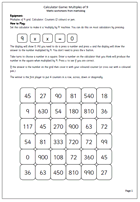It is important that children become familiar with calculators as they will be using them more and more as they progress through High School. There are many skills to learn and in this activity it is certainly a great help to be able to work out mentally division calculations. as the calculator will only confirm or otherwise your calculation.

This is the final game in our calculator series looking at tables and multiples. Once again the idea of the game is to get four counters in a row, this time on a multiples of 9 grid. Recognising multiples of 9 is relatively easy as the digits always add up to 9 or a multiple of 9. But knowing exactly which multiple is needed is much harder. Why not challenge your children?!!

Multiples of 9 calculator game

## Calculator words (upside down)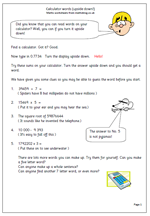As the summer term comes to an end why not have some more fun with a calculator. I expect most children have worked out the certain combinations of letters will form words when the calculator is turned upside down and this page looks a little more closely at this.

For example: 0.7734 will greet you with the word ‘hello’, albeit in a slightly odd font, but perfectly readable.

There follows 5 calculations which give words.

Once children have worked out which letters can be converted into words then there is a tremendous range of words that can be created. The second page suggests just a few of them.

One good idea is to let children find the number that makes a word and then make a clue up , in a similar way to the worksheet. This will involve plenty of excellent practice at working with numbers.

Calculator words

## Year 5 Calculator game: Multiples of 8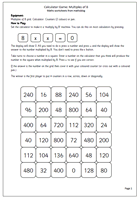This is the next in our series of calculation activities to encourage knowledge of tables. It concentrates on multiples of 8 and like the other games we have published the aim is to cover a row of four squares. Some of the answers are quite tricky to find, such as finding what number multiplied by 8 makes 112?

Remember that the calculator can probably be made into a ‘multiply by 8’ machine by pressing: 8 x x = 0, then all you need to type in is the number and = to find out what 8 times the number is.

Multiples of 8 calculator game

## Calculator game: 7x table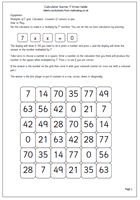Of all the tables, the 7x table is probably the least well known. The latest in our series of calculator games for two players helps with reinforcing the 7x table. Remember, as before, the aim of the game is to get four in a row, in any direction, before your opponent. A good knowledge of the 7x table will help with this.

This sequence of calculator games can be found in Year 3 and Year 4 Knowing Number Facts.

7x table calculator game

## Calculator game: 6x table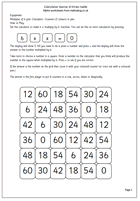The six times table is one of the hardest to learn, but here is another in our calculator games sequence which will help reinforce the table. Remember the idea is to choose a number on the board and then work out which number multiplied by 6 will make the chosen number.

This can be found in our Year 4 resources under Knowing Number Facts.

6x table calculator game

## Calculator game: 5x table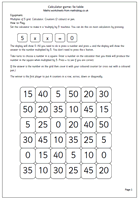This is another in our series of using a calculator to help learn and reinforce tables. To be successful with this game you need to know your 5x table: the calculator just confirms your correct mental arithmetic (or not!). This series of calculator activities for 2x, 3x, 5x and 10x tables are all found in the Year 3: Know number facts section.

## Calculator game: Multiples of 10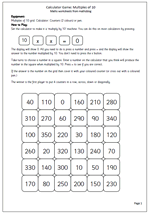Multiples of ten are fairly easy to work out, but nevertheless this is a good game of strategy to play to help reinforce multiples of 10. Choose a number on the grid then try and make it on the calculator by multiplying a number by 10. If correct and the answer on the calculator matches the number on the grid place a coloured counter on the grid. Play then goes to your opponent, with the aim of trying to get 4 in a row.

Calculator game: Multiples of 10

## Calculator game: Multiples of 5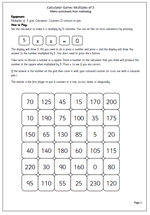The idea of this game is to make a row of 4 in any direction before your opponent. Take it in turns to use the calculator to multiple chosen numbers to match numbers on the grid. This is excellent practice at multiplying and dividing by 5 mentally and then using the calculator to check answers. Suitable for year 3 upwards.

Multiples of 5_larger numbers

## Calculator game: Multiples of 4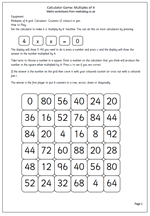Once children have learned the 4 times table this calculator game can be played. Set up the calculator to be a 4 times table machine by pressing 4 x x = 0 and then all you have to do to multiply a number by 4 is to type the number and press equals (don’t delete the answer before pressing the next number). Choose a number on the grid and say it out loud, then try and make that number by entering a number on the calculator and multiplying it by 4 by pressing =. If correct place a counter on the grid. Continue taking in turns with the aim of getting 4 in a row.

Complicated? Why not try the game and see just how easy it is.

Multiples of 4_larger numbers

## Calculator game: 4x table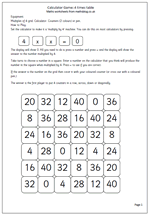Here is another in our series of calculator activities for year 3 children. It is a two player game intended to help with reinforcing the 4 times table. Calculators for young children have been frowned upon over the last few years but they can be a powerful tool for learning and should not be ignored as a resource. We will be following this up with a similar ‘multiples of 4’ page in a couple of weeks time.

4x table calculator game